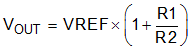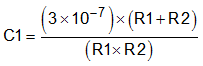ZHCSHI5D March   2001  – February 2018

PRODUCTION DATA.

1. 特性
2. 应用
3. 说明
1.     Device Images
4. 修订历史记录
5. Pin Configuration and Functions
6. Specifications
7. Detailed Description
1. 7.1 Overview
2. 7.2 Functional Block Diagrams
3. 7.3 Feature Description
4. 7.4 Device Functional Modes
8. Application and Implementation
1. 8.1 Application Information
2. 8.2 Typical Application
3. 8.3 Do's and Don'ts
9. Power Supply Recommendations
10. 10Layout
11. 11器件和文档支持
12. 12机械、封装和可订购信息

• DBV|5
• DBV|6

#### 7.3.2 Programming the TPS79101 Adjustable Regulator

The output voltage of the TPS79101 adjustable regulator is programmed using an external resistor divider; see Figure 32. The output voltage is calculated using:

Equation 3.where

• VREF = 1.2246 V typ (the internal reference voltage)

Select resistors R1 and R2 for approximately a 50-µA divider current. Lower value resistors can be used for improved noise performance, but the solution consumes more power. Avoid higher resistor values because leakage current into or out of FB across R1, R2 creates an offset voltage that artificially increases or decreases the feedback voltage and thus erroneously decreases or increases VOUT. The recommended design procedure is to choose R2 = 30.1 kΩ to set the divider current at 50 µA, C1 = 15 pF for stability, and then calculate R1 using:

Equation 4.In order to improve the stability of the adjustable version, a small compensation capacitor is suggested to be placed between OUT and FB. For voltages < 1.8 V, the value of this capacitor must be 100 pF. For voltages > 1.8 V, the approximate value of this capacitor can be calculated as:

Equation 5.The table in Figure 34 shows the suggested value of this capacitor for several resistor ratios. If this capacitor is not used (such as in a unity-gain configuration) or if an output voltage < 1.8 V is chosen, then the minimum recommended output capacitor is 2.2 µF instead of 1 µF.Figure 34. TPS79101 Adjustable LDO Regulator Programming